FundooTutor

# Exercise 9.1

#### 1. A circus artist is climbing a 20 m long rope, which is tightly stretched and tied from the top of a vertical pole to the ground. Find the height of the pole, if the angle made by the rope with the ground level is 30° (see figure ).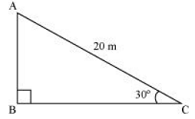Let AB be the vertical pole Ac be 20 m  long rope tied to point C.
In  right ΔABC,
sin 30° = AB/AC
⇒ 1/2 = AB/20
⇒ AB = 20/2
⇒ AB = 10
The height of the pole is 10 m.

2. A tree breaks due to storm and the broken part bends so that the top of the tree touches the ground making an angle 30° with it. The distance between the foot of the tree to the point where the top touches the ground is 8 m. Find the height of the tree.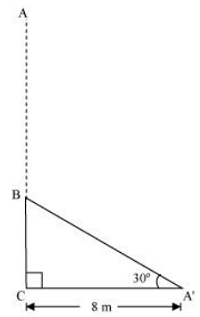Let AC be the broken part of the tree.
∴ Total height of the tree = AB+AC
In  right ΔABC,
cos 30° = BC/AC
⇒ √3/2 = 8/AC
⇒ AC = 16/√3
Also,
tan 30° = AB/BC
⇒ 1/√3 = AB/8
⇒ AB = 8/√3
Total height of the tree = AB+AC = 16/√3 + 8/√3 = 24/√3

3. A contractor plans to install two slides for the children to play in a park. For the children below the age of 5 years, she prefers to have a slide whose top is at a height of 1.5 m, and is inclined at an angle of 30° to the ground, whereas for elder children, she wants to have a steep slide at a height of 3 m, and inclined at an angle of 60° to the ground. What should be the length of the slide in each case?

####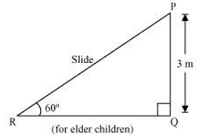In  right ΔPQR, sin 60° = PQ/PR ⇒ √3/2 = 3/PR ⇒ PR = 2√3 m Hence, length of the slides are 3 m and 2√3 m respectively.

4. The angle of elevation of the top of a tower from a point on the ground, which is 30 m away from the foot of the tower, is 30°. Find the height of the tower.

Let AB be the height of the tower and C is the point elevation which is 30 m away from the foot of the tower.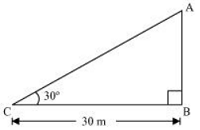#### In  right ΔABC, tan 30° = AB/BC ⇒ 1/√3 = AB/30 ⇒ AB = 10√3 Thus, the height of the tower is 10√3 m.

5. A kite is flying at a height of 60 m above the ground. The string attached to the kite is temporarily tied to a point on the ground. The inclination of the string with the ground is 60°. Find the length of the string, assuming that there is no slack in the string.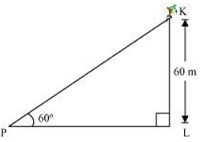Let BC be the height of the kite from the ground,
AC be the inclined length of the string from the ground and A is the point where string of the kite is tied.
A/q,
In  right ΔABC,
sin 60° = BC/AC
⇒ √3/2 = 60/AC
⇒ AC = 40√3 m
Thus, the length of the string from the ground is 40√3 m.

6. A 1.5 m tall boy is standing at some distance from a 30 m tall building. The angle of elevation from his eyes to the top of the building increases from 30° to 60° as he walks towards the building. Find the distance he walked towards the building.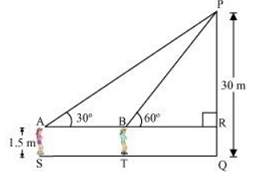Let the boy initially standing at point Y with inclination 30° and then he approaches the building to
the point X with inclination 60°.
∴ XY is the distance he walked towards the building.
also, XY = CD.
Height of the building = AZ = 30 m
AB = AZ – BZ = (30 – 1.5) = 28.5 m
A/q,
In  right ΔABD,
tan 30° = AB/BD
⇒ 1/√3 = 28.5/BD
⇒ BD = 28.5√3 m
also,
In  right ΔABC,
tan 60° = AB/BC
⇒ √3 = 28.5/BC
⇒ BC = 28.5/√3 = 28.5√3/3 m
∴ XY = CD = BD – BC = (28.5√3 – 28.5√3/3) = 28.5√3(1-1/3) = 28.5√3 × 2/3 = 57/√3 m.
Thus, the distance boy walked towards the building is 57/√3 m.

7. From a point on the ground, the angles of elevation of the bottom and the top of a transmission tower fixed at the top of a 20 m high building are 45° and 60° respectively. Find the height of the tower.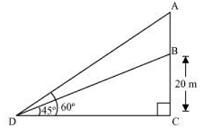Let BC be the 20 m high building.
D is the point on the ground from where the elevation is taken.
Height of transmission tower = AB = AC – BC
In  right ΔBCD,
tan 45° = BC/CD
⇒ 1 = 20/CD
⇒ CD = 20 m
also,
In  right ΔACD,
tan 60° = AC/CD
⇒ √3 = AC/20
⇒ AC = 20√3 m
Height of transmission tower = AB = AC – BC = (20√3 – 20) m = 20(√3 – 1) m.

8. A statue, 1.6 m tall, stands on the top of a pedestal. From a point on the ground, the angle of elevation of the top of the statue is 60° and from the same point the angle of elevation of the top of the pedestal is 45°. Find the height of the pedestal.